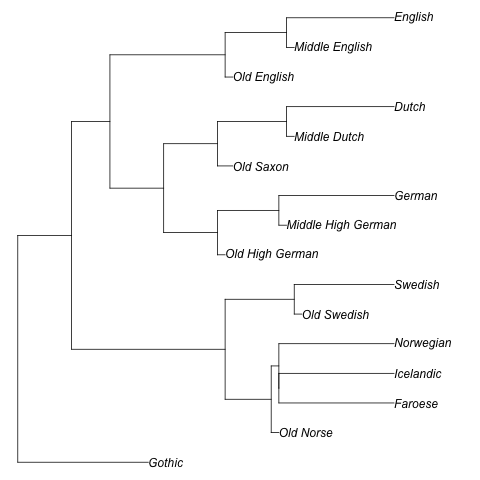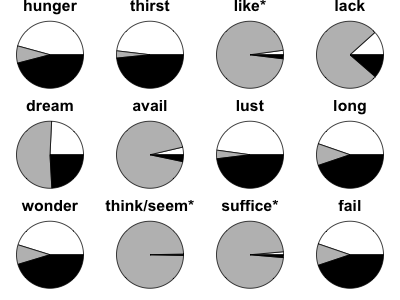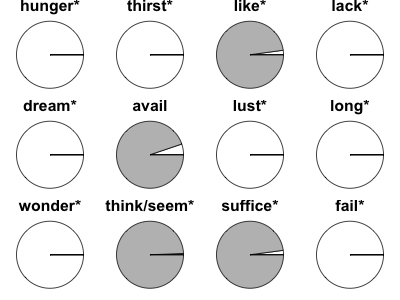# Dative Sickness¶

This jupyter notebook contains all the information necessary to replicate the study in Dative Sickness: A Phylogenetic Analysis of Argument Structure Evolution in Germanic. The notebook and supporting files are available as a repository on GitHub: https://github.com/evoling/dative-sickness. A static (read-only, non-interactive) version can be found at http://evoling.github.io/dative-sickness/.

## 0. Setup¶

Before we start, check that necessary tools are present, and that we can find all the necessary data files. Click on the box below to select it and press the Run Cell button (the rightward-facing triangle). The selection automatically progresses to the next cell, so you can work through the notebook by pressing Run Cell repeatedly.

In :
# Is BayesTraits installed?
from subprocess import check_output
from hashlib import md5
import rpy2
# this will raise a CalledProcessError if BayesTraits isn't installed and on the path
BAYESTRAITS = check_output(["which", "BayesTraits"]).strip()
# document which version of the binary we are using
print("Binary version (md5 hash):", md5(open(BAYESTRAITS, "rb").read()).hexdigest())

Binary version (md5 hash): 0b6a340317053e4cbf7ad86e059ded43


This notebook is written in python version 3, and requires the jupyter and rpy2 libraries and their dependencies, which can be installed using the command pip install jupyter rpy2. The rpy2 library is a bridge to the R statistical language, which should also be installed, along with the R library ape (installed within R using install.packages("ape")). The workflow assumes a unix-like system, such as MacOSX or Linux.

In :
# Are the data files present
from os.path import exists
DATAFILE = "data/GmcArgStrucStates.csv"
assert exists(DATAFILE)
TREEFILE = "data/germanic-tree-2.tre"
assert exists(TREEFILE)

In :
# Setup for using R through python
%Rpush TREEFILE

In :
%%R
# Check the reference tree
library("ape")## 1. MULTISTATE test: All Models¶

This test runs a multistate analysis of every possible model of evolution, defined by constraining a subset of the transition probabilities to zero. The unconstrained model (i.e. the model which estimates all transitions) is:

A N D
N qNA qND
D qDA qDN

The Dative sickness model is defined as:

A N D
N 0 0
D 0 qDN

The following snippet creates BayesTraits command files for all possible models, as well a shell script RUNFILE to run them. This produces a MULTISTATE analysis using Maximum Likelihood. Testing showed that 1000 repeats of the ML test ensures a stable response.

In :
from itertools import chain, combinations
RUNFILE = "build/runfile.sh"

transitions = ["q"+i+j for i in "ADN" for j in "ADN" if i != j]

def all_subsets(ss):
return chain([None], *map(lambda x: combinations(ss, x), range(1, len(ss))))

with open(RUNFILE, "w") as runfile:
for i, subset in enumerate(all_subsets(transitions)):
filename = "build/job{:02d}.cmd".format(i)
print(BAYESTRAITS, TREEFILE, DATAFILE, "<", filename, "> /dev/null",
file=runfile)
with open(filename, "w") as fo:
print("1", file=fo) # MULTISTATE analysis type
print("1", file=fo) # Maximum likelihood
print("logfile build/job{:02d}.log".format(i), file=fo)
print("mltries 1000", file=fo) # Run ML 1000x to ensure a stable response
if subset: # else unconstrained model
subset += ("0",)
print("restrict", *subset, file=fo)
print("run", file=fo)
print("echo All log files are complete!", file=runfile)


The next command could take a few minutes. Do not continue further until all the log files are complete. Note that the prompt will have a star instead of a number while a script is still processing, like this: In [*]

In :
%%script bash
sh ./build/runfile.sh

[?7h[4l>7[r[?1;3;4;6l8All log files are complete!

###### Summarise models¶
In :
from glob import glob
from io import StringIO
from IPython.display import HTML

"Root - S(0) - P(A)", "Root - S(0) - P(D)", "Root - S(0) - P(N)",
"Root - S(1) - P(A)", "Root - S(1) - P(D)", "Root - S(1) - P(N)",
"Root - S(2) - P(A)", "Root - S(2) - P(D)", "Root - S(2) - P(N)",
"Root - S(3) - P(A)", "Root - S(3) - P(D)", "Root - S(3) - P(N)",
"Root - S(4) - P(A)", "Root - S(4) - P(D)", "Root - S(4) - P(N)",
"Root - S(5) - P(A)", "Root - S(5) - P(D)", "Root - S(5) - P(N)",
"Root - S(6) - P(A)", "Root - S(6) - P(D)", "Root - S(6) - P(N)",
"Root - S(7) - P(A)", "Root - S(7) - P(D)", "Root - S(7) - P(N)",
"Root - S(8) - P(A)", "Root - S(8) - P(D)", "Root - S(8) - P(N)",
"Root - S(9) - P(A)", "Root - S(9) - P(D)", "Root - S(9) - P(N)",
"Root - S(10) - P(A)", "Root - S(10) - P(D)", "Root - S(10) - P(N)",
"Root - S(11) - P(A)", "Root - S(11) - P(D)", "Root - S(11) - P(N)"]

data = {}

for filename in glob("build/*.log"):
restriction0 = set()
with open(filename) as fileobj:
for line in fileobj:
if line.startswith("Restrictions"):
break
# parse restrictions
counter = 0
for line in fileobj:
counter += 1
row = line.strip().split()
try:
float(row)
except ValueError:
pass
if counter == 6:
break
model = "=".join(sorted(restriction0)+["0"])
for line in fileobj:
pass
# parse final line
row = [float(e) for e in line.strip().split()]
row_data = {}
for key, value in zip(header, row):
if key.startswith("q") or key == "Lh":
row_data[key] = value
elif key.startswith("Root"):
lexeme_key = key.split()
state_key = key.split()[-1]
row_data.setdefault(lexeme_key, {})[state_key] = value
if model == "0":
Lh_unconstrained = row_data["Lh"]
data[model] = row_data

lexemes = ["hunger", "thirst", "like", "lack", "dream", "avail",
"lust", "long", "wonder", "think/seem", "suffice", "fail"]
CM_recon = ["A", "A", "D", "A", "A", "D", "A", "A", "A", "D", "D", "A"]

["{} *{}".format(l, r) for l, r in zip(lexemes, CM_recon)] +
["mismatch", "Lh_ratio"])

results = StringIO()

print("<table>", file=results)
print("<tr>{}</tr>".format(
file=results)

def get_name(model):
if model == "qDA=qNA=qND=0":
return model + " (Dative Sickness)"
return model + " (Accusative Sickness)"
elif model == "qDA=qDN=qND=0":
return model + " (Nominative Sickness)"
elif model == "0":
return "Unconstrained"
else:
return model

rows = []
for model in sorted(data):
row_data = data[model]
row = [get_name(model)]
for key in ["Lh", "qAD", "qAN", "qDA", "qDN", "qNA", "qND"]:
row.append(row_data[key])
mismatch = 0
for i, cm in zip(range(14), CM_recon):
try:
d = row_data["S({})".format(i)]
recon = sorted(d, key=lambda k: d[k])[-1]
if d[recon] < 0.95:
recon = "?"
mismatch += 1
else:
recon = recon[-2]
if recon != cm:
mismatch += 1
row.append(recon)
except KeyError:
pass
row.append(mismatch)
row.append(2* (Lh_unconstrained - row_data["Lh"]))
rows.append(row)

for row in sorted(rows, key=lambda e: (e!="Unconstrained", -e)):
print("<tr>{}</tr>".format(
"".join(["<td>{}</td>".format(item) for item in row])),
file=results)
print("</table>", file=results)

results.seek(0)

Out:
modelLhqADqANqDAqDNqNAqNDhunger *Athirst *Alike *Dlack *Adream *Aavail *Dlust *Along *Awonder *Athink/seem *Dsuffice *Dfail *AmismatchLh_ratio
Unconstrained-104.5794040.0001180.0068130.00.0004620.0085475.8e-05??D??????DD?90.0
qDA=0-101.4846590.0001260.0009320.00.0004390.0012535.1e-05??D??????DD?9-6.189490000000006
qDA=qND=0-101.4901330.000150.0009220.00.0004340.0012850.0??D??????DD?9-6.178541999999993
qND=0-101.4901550.000150.0009240.00.0004340.0012890.0??D??????DD?9-6.1784979999999905
qDN=qND=0-105.1173210.0001470.485270.0004680.00.5876190.0??D??????DD?91.0758340000000146
qDN=0-105.1349760.0001270.1058830.0004630.00.1258512.5e-05??D??????DD?91.111143999999996
qNA=0-106.5718460.00.0006289e-050.0005970.00.001045AA????AA???A73.984883999999994
qDA=qNA=0-107.44990.00.0006230.00.0006590.00.001122AA?AA?AAA??A45.740992000000006
qAN=qDA=0-112.3966080.0007020.00.02.2339220.0002762.863408AA????A?????915.634408000000008
qAN=qNA=0-112.4032110.0006860.00.000220.7651140.00.973112AA????A?????915.647614000000004
qAN=0-112.4320660.0006980.00.00.1433960.0002720.172037AA????A?????915.705324000000019
qAN=qDA=qNA=0-115.1502680.0006730.00.09.3668660.012.012797AA?AA?AAA??A421.141728
qDN=qNA=0-117.7702656.5e-050.000820.0005690.00.08.7e-05?????????DD?1026.381721999999996
qDN=qNA=qND=0-118.5103196.5e-050.0008080.0005870.00.00.0??DD?D???DD?827.861829999999998
qAN=qND=0-120.8063450.0022960.00.0010570.0012910.0011990.0????????????1232.45388199999999
qAN=qDA=qND=0-123.7710250.0006940.00.00.0011956.6e-050.0AA????AA???A738.383241999999996
qAN=qNA=qND=0-126.5268240.0007920.00.0001160.0008250.00.0AA????AA???A743.894840000000016
qAN=qDA=qNA=qND=0-128.0912170.0007540.00.00.0008580.00.0AA?AA?AAA??A447.02362600000001
qDA=qDN=0-156.7371430.00.0007980.00.00.0003750.000804AA????A?????9104.31547800000001
qDA=qDN=qNA=0-159.7495125e-060.0006310.00.00.00.000917AA?AA?AAA??A4110.34021600000003
qDA=qDN=qND=0 (Nominative Sickness)-162.7220970.0005680.1815140.00.00.2292360.0????????????12116.28538599999999
qDA=qDN=qNA=qND=0-183.299760.0003880.000520.00.00.00.0AAAAAAAAAAAA4157.440712
qAN=qDN=0-255.4160870.0002580.00.00.00.0005040.000408NNNNNNNNNNNN12301.673366
qAN=qDA=qDN=0-255.416090.0002580.00.00.00.0005040.000408NNNNNNNNNNNN12301.673372
qAN=qDN=qNA=0-261.2541880.0969090.00.1148370.00.00.000912NNNNNNNNNNNN12313.349568
qAN=qDN=qND=0-261.3193837.6943020.08.4866960.00.0009140.0NNNNNNNNNNNN12313.479958
qAN=qDA=qDN=qND=0-281.0112210.0019480.00.00.00.0009140.0NNNNNNNNNNNN12352.86363399999993
qAN=qDA=qDN=qNA=0-825.214450.0001610.00.00.00.00.0007AANANNAANNNA61441.2700920000002
qAN=qDA=qDN=qNA=qND=0-1779.5153880.0243440.00.00.00.00.0NN????AAN??A93349.871968
###### Make Pie Data¶

This snippet creates two data files containing ancestral state reconstruction probabilities for the Unconstrained and Dative sickness models

In :
from __future__ import print_function

template = 'Root - S({N}) - P({state})'

for filein, fileout in [
("build/job00.log", "build/asr-unconstrained.csv"),
("build/job40.log", "build/asr-dative-sickness.csv")]:
with open(filein) as logfile:
with open(fileout, "w") as outfile:
keys = lines[-2].strip().split("\t")
values = map(float, lines[-1].strip().split("\t"))
data = dict(zip(keys, values))
for N in range(12):
row = [lexemes[N]]
key = template.format(N=N, state=state)
row.append(data[key])
if state == CM_recon[N]:
if data[key] > 0.95:
row += "*"
print(*row, sep="\t", file=outfile)

###### Plot pies¶

Plot the data from the files generated above.

In :
%%R
# library(RColorBrewer)
# colours <- brewer.pal(3, "Dark2")
colours <- c("white", "gray", "black")

png("build/asr-unconstrained.png", width=400, height=300)
par(mfrow=c(3,4), mar=c(0,0,1,0))
for (i in 1:12)
pie(unlist(d[i,]), labels=NA, col=colours,
main=row.names(d)[i], cex.main=2)
. <- dev.off()

png("build/asr-dative-sickness.png", width=400, height=300)
par(mfrow=c(3,4), mar=c(0,0,1,0))
for (i in 1:12)
pie(unlist(d[i,]), labels=NA, col=colours,
main=row.names(d)[i], cex.main=2)
. <- dev.off()

In :
from IPython.display import Image
Image(filename="build/asr-unconstrained.png")

Out:In :
Image(filename="build/asr-dative-sickness.png")

Out:In [ ]:


In [ ]: